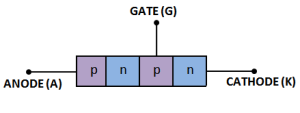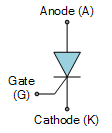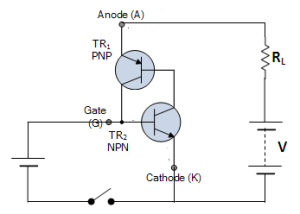# Silicon Controlled Rectifier (SCR) | Construction & Working

## Silicon Controlled Rectifier (SCR)

In this article we are going to discuss the construction and working of an silicon controlled rectifier (SCR) in detail.

The silicon controlled rectifier ( SCR ) is a three terminal semiconductor switching device which can be used as a controlled switch to perform various functions such as rectification, inversion and regulation of power flow.

An SCR can handle currents upto several thousand amperes and voltages upto more than 1kV.

The SCR has appeared in the market under different names such as thyristor, thyrode transistor.

Like the diode, SCR is a unidirectional device,i.e. it will only conduct current in one direction only, but unlike a diode, the SCR can be made to operate as either an open-circuit switch or as a rectifying diode depending upon how its gate is triggered.

In other words, SCR can operate only in the switching mode and cannot be used for amplification.

Hence, it is extensively used in switching d.c. and a.c., rectifying a.c. to give controlled output, converting d.c. into a.c. etc.

### Constuctional Details of SCR

When a pn junction is added to a junction transistor, the resulting three pn junctions device is called a silicon controlled rectifier.

Fig.1(i) shows the construction of an SCR.Fig.1 (i)

It is clear that it is essentially an ordinary rectifier (pn) and a junction transistor  (npn) combined in one unit to form pnpn device.

Three terminals are taken; one from the outer p-type material called anode A, second from the outer layer of n-type material called cathode K and the third from the base of transistor section and is called gate G.

In the normal operating conditions of SCR, anode is held at high positive potential w.r.t. cathode and gate at small positive potential w.r.t. cathode.

Fig.1 (ii) shows the symbol of SCR.Fig.1 (ii)

### Working of SCR

In a silicon controlled rectifier, load is connected in series with anode.

The anode is always kept at positive potential w.r.t. cathode.

The working of SCR can be studied under the following two heads:

#### 1. When gate is open:

Fig.2 shows the SCR circuit with gate open i.e. no voltage applied to the gate.

Fig.2

Under this condition, junction J2 is reverse biased while junction J1 and J3 are forward biased.

Hence, the situation in the junctions J1 and J3 is just as in a npn transistor with base open.

Consequently, no current flows through the load RL and the SCR is cut off.

However, if the applied voltage is gradually increased, a stage is reached when the reverse biased junction J2 breaks down.

The SCR now conducts heavily and is said to be in the ON state.

The applied voltage at which SCR conducts heavily without gate voltage is called Breakover voltage.

#### 2. When gate is positive w.r.t. cathode

The SCR can be made to conduct heavily at smaller applied voltage by applying a small positive potential to the gate as shown in fig.3.

Fig.3

Now junction J3 is forward biased and junction J2 is reverse biased.

The electrons from n-type material start moving across junction J3 towards left whereas holes from p-type towards the right.

Consequently, the electrons from junction J3 are attracted across the junction J2 and gate current starts flowing .

As soon as the gate current flows, anode current increases.

The increased current in turn makes more electrons available at junction J2.

This process continues and in an extremely small time, junction J2 breaks down and the SCR starts conducting heavily.

Once SCR starts conducting, the gate loses all control. Even if gate voltage is removed, the anode current does not decrease at all.

The only way to stop conduction i.e. to bring the SCR in off condition, is to reduce the applied voltage to zero.

#### Conclusion

The following conclusions are drawn from the working of SCR:

1. An SCR has two states i.e. either it does not conduct or it conducts heavily. There is no state inbetween. Therefore, SCR behaves like a switch.
2. There are two ways to turn on the SCR. The first method is to keep the gate open and make the supply voltage equal to the breakover voltage. The second method is to operate SCR with supply voltage less than breakover voltage and then turn it on by means of a small voltage applied to the gate.
3. Applying small positive voltage to the gate is the normal way to close an SCR because the breakover voltage is usually much greater than supply voltage.
4. To open the SCR e. to make it non-conducting, reduce the supply voltage to zero.

### Equivalent Circuit of SCR

The SCR shown in fig.4 (i) can be visualized as separated into two transistors as shown in fig.4 (ii).

(i)                                                                         (ii)                                                                        (iii)

Fig.4

Thus, the equivalent circuit of SCR is composed of pnp transistor and npn transistor connected as shown in fig 4.(iii).

It is clear that collector of each transistor is coupled to the base of of the other, thereby making a positive feedback loop.

The working of SCR can be easily explained from its equivalent circuit.

Fig.5 shows the equivalent circuit of SCR with supply voltage V and load resistance RL.Fig.5

Assume the supply voltage V is less than breakover voltage as is usually the case.

With gate open (i.e. switch S open), there is no base current in transistor TR2. Therefore, no current flows in the collector of TR2 and hence that of TR1.

Under such conditions, the SCR is open.

However, if the switch S is closed, a small gate current will flow through the base of TR2 which means its collector current will increase.

The collector current of TRis the base current of TR1. Therefore, collector current of TR1 increases.

But collector current of TR1 is the base current of TR2. This action is accumulative since an increase of current in one transistor causes an increase of current in the other transistor.

As a result of this action, both transistors are driven into saturation, and hence heavy current flows through the load RL.

Under such conditions, the SCR closes.

You Might Like The Following Articles